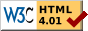## Dokumentation zu: last_bit(E)``` SYNOPSIS int last_bit(string str) DESCRIPTION Return the number of the last set bit in bitstring . Each character contains 6 bits. So you can store a value between 0 and 63 in one character (2^6=64). Starting character is the blank " " which has the value 0. The first character in the string is the one with the lowest bits (0-5). EXAMPLES string s; s = set_bit("", 4); s = set_bit(s, 2); last_bit(s) --> returns 4 SEE ALSO set_bit(E), clear_bit(E), next_bit(E), test_bit(E), count_bits(E), and_bits(E), or_bits(E), xor_bits(E), invert_bits(E), copy_bits(E) ``` Die Seite ist auch in Deutsch vorhanden.

Start » Magierhandbuch » Docu » Efun » Last_bit Letzte Generierung: 25.04.2021, 01:58mud@wl.mud.de